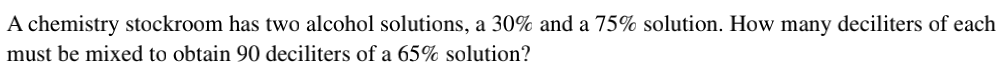### ¿Todavía tienes preguntas de matemáticas?

Pregunte a nuestros tutores expertos
Algebra
PreguntaA chemistry stockroom has two alcohol solutions, a $$30 \%$$ and a $$75 \%$$ solution. How many deciliters of each must be mixed to obtain $$90$$ deciliters of a $$65 \%$$ solution?

let the quantity of 30% of alcohol be x

and let the quantity of 75% of alcohol be y

x+y=90

0.3x+0.75y=90(0.65) = 58.5

x=20 deciliters

y=70 deciliters

Solución
View full explanation on CameraMath App.